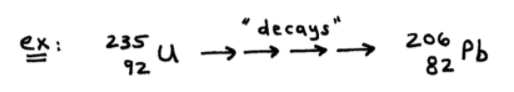# S19E2 - Nuclear Reactions and Radioactive Decay

There are two main categories of radioactive decay:

1. Those that DO involve a change in the mass number (A) of the decaying nucleus, and...

➞ ex:  Alpha-Particle Production
➞ ex:  Spontaneous Fission

2. Those that DO NOT.

➞ ex:  Beta-Particle Production
➞ ex:  Gamma Ray Production
➞ ex:  Electron Capture
➞ ex:  Positron Production

-----

First, we'll examine the 1st category -- those nuclear decay reactions that DO involve a change in the mass number (A).

### Alpha-Particle Production

An alpha-particle (α-particle) is just a helium nucleus, containing 2 protons and 2 neutrons. No electrons!Here are two example alpha-particle decay reactions:-----

### Spontaneous Fission

Fission = the splitting of a heavy nuclide into two lighter nuclides with similar (but smaller) mass numbers. See Californium-254 here...-----

Now, let's examine the 2nd category -- those nuclear decay reactions that DO NOT involve a change in the mass number (A).

### Beta-Particle Production

A beta-particle (β-particle) is just an electron and is often represented as either "e-" or "β-"

In a β-decay reaction, an electron is produced and the mass number of the decaying nucleus remains unchanged. See the beta-decays of Thorium-234 and Iodine-131 below...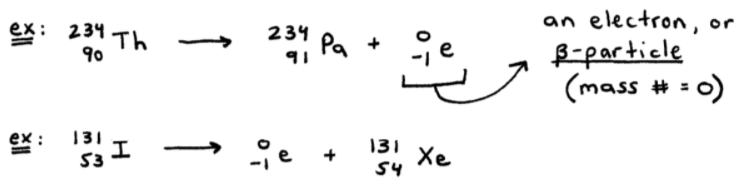-----

### Gamma-Ray Production

Gamma-rays are produced when a high-energy (excited) nucleus loses energy in the form of electromagnetic radiation (EMR) called γ-rays.

In the gamma-decay reaction below, an excited Thorium-230* relaxes back down to its ground state by discarding or "giving off" a photon (γ-ray)...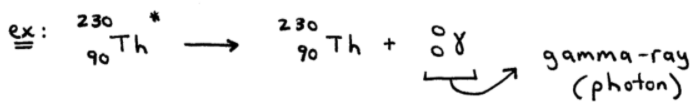-----

### Electron Capture

Electron capture is the opposite of beta-particle production.

Instead of producing an electron, the nuclide (ex: mercury-201) adds or "captures" an electron to form new products:-----

### Positron Production

Positron = a beta+ particle, written as shown below (sometimes "e+" is replaced with "β+")Positron emission is commonly encountered with artificially produced radioactive nucleii of lighter elements.  Phosphorus-30 shows how this decay reaction proceeds...=====

NOTE - Sometimes nuclear decays and particle reactions, such as alpha-particle decay, are accompanied by gamma-ray production.

As first-year general chemistry students, we usually must be told when/if gamma rays are produced.

Why? Because with both A = 0 and Z = 0, it's too difficult to just "know" if a gamma ray is "present" as a product or not.  See here...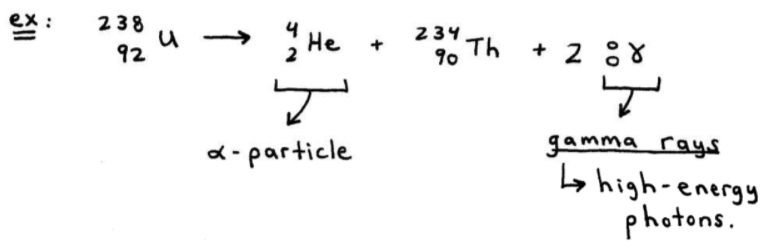=====

## Writing and Balancing Nuclear Reactions

Balance the following nuclear decay reactions:

ex:   Bismuth-214 produces (emits) a beta-particle. Assume only two products.-----

ex:   Neptunium-237 produces (emits) an alpha-particle. Assume only two products.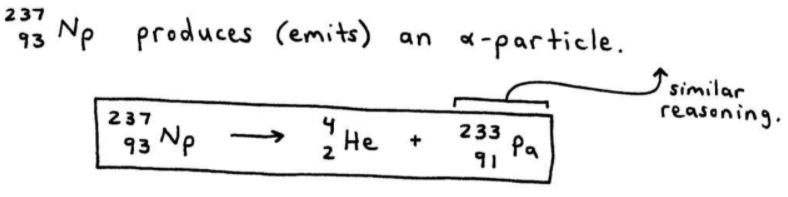-----

ex:   Fill In.  Gold-195  +  [ ? ]    ➞  Platinum-195.The key to doing the above electron capture problem, is to remember that A (top) and Z (bottom) values must be conserved.

=====